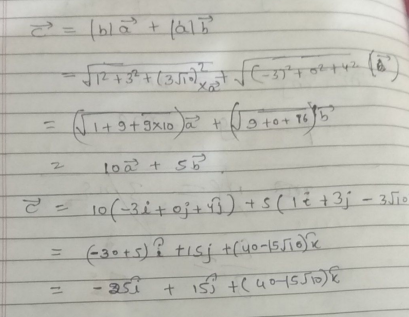### ¿Todavía tienes preguntas de matemáticas?

Pregunte a nuestros tutores expertos
Algebra
Pregunta2. Let vectors $$\vec { a }$$ and $$\vec { b }$$ are placed tail to tail. Find a vector $$\vec { c }$$ in the same direction as a bisectrix of the angle between $$\vec { a }$$ and $$\vec { b }$$ , if:

$$\vec { a } = ( - 3 ; 0 ; 4 ) , \vec { b } = ( 1 ; 3 ; - 3 \sqrt { 10 } )$$